Perpendicular to a line through a point not on it

Chapter 14 Class 6 Practical Geometry
Concept wise

Let’s take an example

1. Given a line l
with point P not on it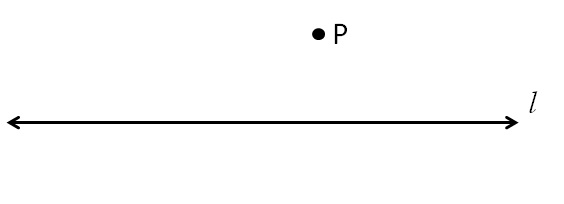2.Place set square on the line so that line is exactly above the set-square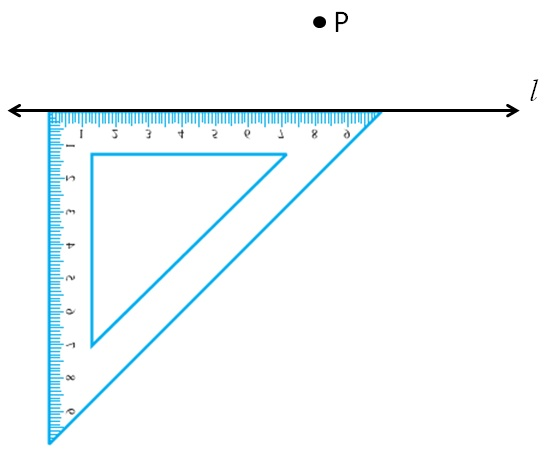1. Put ruler on the hypotenuse side of the set square2. Keeping the ruler in the same position,
slide the set-square till one end of the set-square passes through point P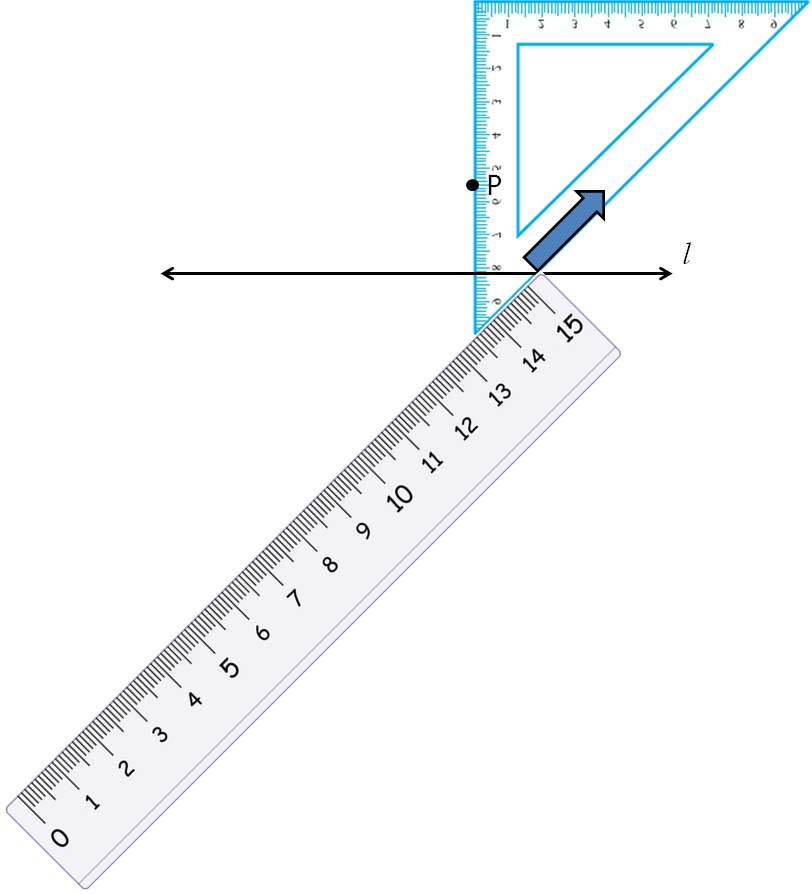3.  Draw a line along the edge of the set square, keeping set-square firm
Let the line intersect line l at Q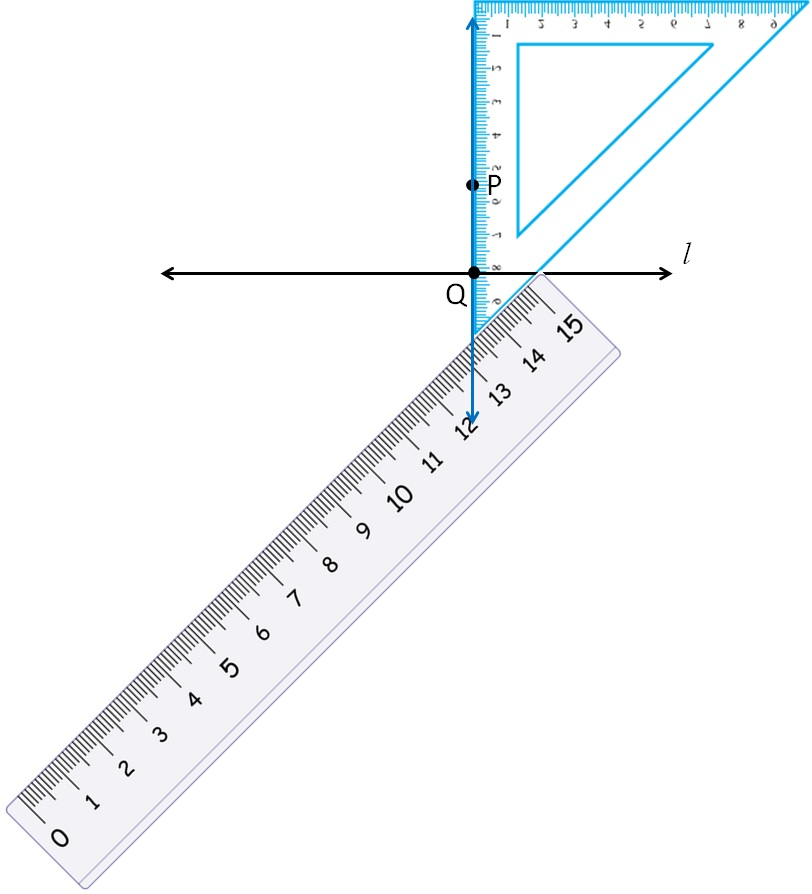Thus, PQ is the perpendicular to l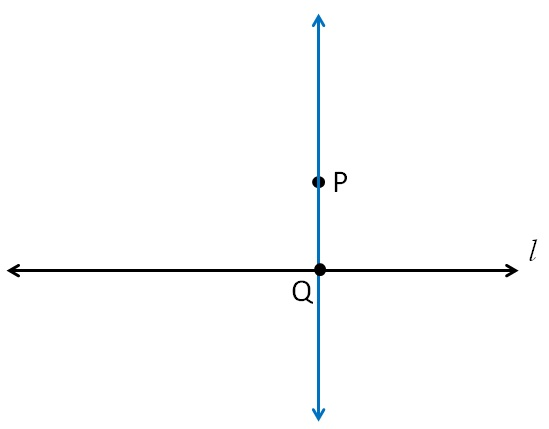Get live Maths 1-on-1 Classs - Class 6 to 12